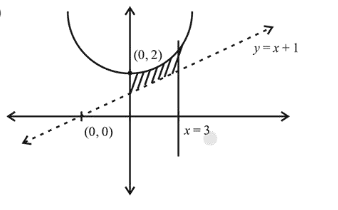# The area (in sq. units) of the region bounded by the parabola,

Question:

The area (in sq. units) of the region bounded by the parabola, $y=x^{2}+2$ and the lines, $y=x+1, x=0$ and $x=3$, is :

1. (1) $\frac{15}{4}$

2. (2) $\frac{21}{2}$

3. (3) $\frac{17}{4}$

4. (4) $\frac{15}{2}$

Correct Option: , 4

Solution:Area of the bounded region $\int_{0}^{3}\left[\left(x^{2}+2\right)-(x+1)\right] d x$

$=\left[\frac{x^{3}}{3}-\frac{x^{2}}{2}+x\right]_{0}^{3}$

$=9-\frac{9}{2}+3=\frac{15}{2}$